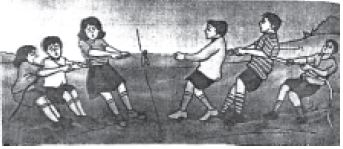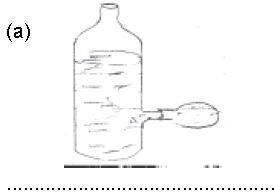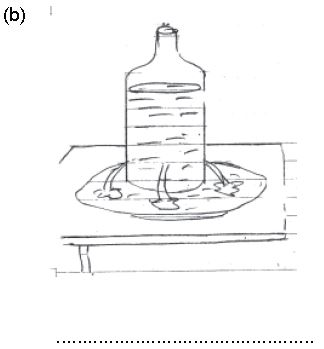# CBSE Class 8 Science Force And Pressure Worksheet

Read and download free pdf of CBSE Class 8 Science Force And Pressure Worksheet. Students and teachers of Class 8 Science can get free printable Worksheets for Class 8 Science Chapter 11 Force and Pressure in PDF format prepared as per the latest syllabus and examination pattern in your schools. Class 8 students should practice questions and answers given here for Science in Class 8 which will help them to improve your knowledge of all important chapters and its topics. Students should also download free pdf of Class 8 Science Worksheets prepared by school teachers as per the latest NCERT, CBSE, KVS books and syllabus issued this academic year and solve important problems with solutions on daily basis to get more score in school exams and tests

## Worksheet for Class 8 Science Chapter 11 Force and Pressure

Class 8 Science students should refer to the following printable worksheet in Pdf for Chapter 11 Force and Pressure in Class 8. This test paper with questions and answers for Class 8 will be very useful for exams and help you to score good marks

### Class 8 Science Worksheet for Chapter 11 Force and Pressure

1) Write one word for the following sentences.

a) A pull or a push on an object is called ______________.

b) A force exerted by a magnet ______________________.

c) A force acting on a unit area of a surface ______________.

d) A pressure exerted by air ___________________.

2) Suppose a man is standing behind a car. Does the man exert the force on the car?3) If the members of two teams, in a game of tug-of-war, are trying to pull the rope in their respective directions and the rope does not move. Which team pulls with more force.4) In the figures shown below, what conclusion can you draw about the pressure exerted by liquids.5. A forced exerted by a magnet on another magnet is
a) contact force
b) Frictional force
c) Non contact force
d) Muscular force

6) The weight of air in a column of the height of the atmosphere and area 10cm x 10cm is as large as
a) 1 Kg.
b) 10 kg.
c) 100 kg.
d) 1000 kg.

7) When air is filled in a container, air
a) Exert pressure at the bottom
B) Exert pressure on upper side.
c) Exert pressure on the walls
d) Non of these

8) The shoulder bags are provided with broad straps.
a) To decrease the pressure
b) To exert more force
c) To increase the pressure
d) None of these.

1. S.I unit of pressure is ____________.

2. The amount or the strength of force is called its_______________.

3. Pressure in solid depends upon ________________ and ____________.

4. Pressure in liquids depends upon _____________ and ______________.

5. Force generated by moving of non living materials is called ______________.

6. Give 2 examples of contact forces.

7. Name the scientist who invented a pump to extract air from a vessel.

8. Name the force which acts upon another body without any connector.

9. Name the type of force involved in the following.
a) To pick your school bag.
b) To collect scrap iron from garbage.
c) To push or pull a loaded trolley ay supermarket.
d) Flying of seeds away from each other in plastic bag when gently rubbed.

10. Name the device used to measure pressure in fluids.

11. How much force is required to lift a mass of 1Kg (10N/1N).

12. A rolling ball stops after sometime due to (gravitational force/ Frictional force).

13. When 2 forces are applied at the same point but in opposite directions the net force is equalto the (sum/ difference) of forces acting separately.

14. Force used to stretch the spring is (muscular force/ magnetic force)

15. Application of force brings the change in
a) shape and size of object
(b) speed and direction of object
(c) position of object
(d) all the above

Q1) Fill in the blanks

a. A force arises due to __________ between two objects.

b. An example of a non-contact force is __________.

c. The pressure exerted by air around us is known as __________.

d. Strength of the force is expressed by its __________.

e. Application of force can change the __________, __________ and __________ of an object.

f. Force exerted by our muscles is called __________ force.

g. Direction of force of friction is always __________ to the direction of motion.

h. Force per unit area is called ___________.

Q2) Complete the following sentences:
a. If two equal forces act in the opposite directions on an object, the net force acting on it is __________.
b. It is easier to cut with a sharp knife than with a blunt one because _________ ____________.
c. Porters place a round piece of cloth on their heads, when they have to carry heavy loads because ____________ _____________

Q3) Give four effects of force. Write one example of each.

Q4) Name
a) the force exerted by all matter on all other matter
b) the force which tends to slow down objects or keep them from moving
c) the force with which a charged body attracts an uncharged body.

Q5) An example of a contact force is
1) Magnetic force
2) Frictional force
3) Gravitational force
4) All of these

Q6) A Force cannot change the
1) Speed of an object
2) Mass of an object
3) Shape of an object
4) The direction of a moving object

Q7) The pull of the earth is a force called
a) Gravitational force
b) Frictional force
c) Electrostatic forces
d) None of these

Q8) If a force is applied on a smaller area of contact, the pressure exerted by it is
1) Greater
2) Lesser
3) The same
4) None of these

Q9) Why do the pillars holding the track of Metro trains have a broader base?

Q10) Define Pressure. Explain why shoulder bags are provided with broad straps and not thin strap.

Q11) Describe an activity to show that
(i) the pressure exerted by a liquid at the bottom of the container, depends on the height of its column.
(ii) liquids exert pressure on the walls of the container.
(iii) liquids exert equal pressure at the same depth.

Q12) Explain why we do not feel the atmospheric pressure.

Q 13) A ball rolling on the ground comes to rest after some time. Name the force involved.

Q 14) Explain the following types of forces. Give one example where each of these could be found operating:
a. Magnetic force c) Electrostatic force
b. Gravitational force d) Frictional force

Q15) Bhola was impressed by the miracle performed by baba Shyamdev, when Baba lied down on a bed of nails. The nails did not hurt him. Bhola narrated this miracle to his friend Rahul. Rahul explained to Bhola that, there is no magic or miracle here. Even he can do the same trick.
(a) Why did Rahul say that , there is no miracle in this trick? Why did Baba Shaymdev not hurt himself?
(b) Which quality is shown by Rahul here?

 CBSE Class 8 Science Crop Production And Management Worksheet
 CBSE Class 8 Science Micro Organisms Friend And Foe Worksheet
 CBSE Class 8 Science Synthetic Fibers And Plastics Worksheet
 CBSE Class 8 Science Materials Metals And Non Metals Worksheet
 CBSE Class 8 Science Coal And Petroleum Worksheet
 CBSE Class 8 Science Combustion And Flame Worksheet
 CBSE Class 8 Science Conservation Of Plants And Animals Worksheet
 CBSE Class 8 Science Cell Structure And Functions Worksheet
 CBSE Class 8 Science Reproduction In Animals Worksheet
 CBSE Class 8 Science Reaching The Age Of Adolescence Worksheet
 CBSE Class 8 Science Force And Pressure Worksheet
 CBSE Class 8 Science Friction Worksheet
 CBSE Class 8 Science Sound Worksheet
 CBSE Class 8 Science Chemical Effect Of Electric Current Worksheet
 CBSE Class 8 Science Some Natural Phenomena Worksheet
 CBSE Class 8 Science Light Worksheet
 CBSE Class 8 Science Stars And The Solar System Worksheet
 CBSE Class 8 Science Pollution Of Air And Water Worksheet

## More Study Material

### CBSE Class 8 Science Chapter 11 Force and Pressure Worksheet

We hope students liked the above worksheet for Chapter 11 Force and Pressure designed as per the latest syllabus for Class 8 Science released by CBSE. Students of Class 8 should download in Pdf format and practice the questions and solutions given in the above worksheet for Class 8 Science on a daily basis. All the latest worksheets with answers have been developed for Science by referring to the most important and regularly asked topics that the students should learn and practice to get better scores in their class tests and examinations. Studiestoday is the best portal for Class 8 students to get all the latest study material free of cost.

### Worksheet for Science CBSE Class 8 Chapter 11 Force and Pressure

Expert teachers of studiestoday have referred to the NCERT book for Class 8 Science to develop the Science Class 8 worksheet. If you download the practice worksheet for one chapter daily, you will get higher and better marks in Class 8 exams this year as you will have stronger concepts. Daily questions practice of Science worksheet and its study material will help students to have a stronger understanding of all concepts and also make them experts on all scoring topics. You can easily download and save all revision worksheet for Class 8 Science also from www.studiestoday.com without paying anything in Pdf format. After solving the questions given in the worksheet which have been developed as per the latest course books also refer to the NCERT solutions for Class 8 Science designed by our teachers

#### Chapter 11 Force and Pressure worksheet Science CBSE Class 8

All worksheets given above for Class 8 Science have been made as per the latest syllabus and books issued for the current academic year. The students of Class 8 can be rest assured that the answers have been also provided by our teachers for all worksheet of Science so that you are able to solve the questions and then compare your answers with the solutions provided by us. We have also provided a lot of MCQ questions for Class 8 Science in the worksheet so that you can solve questions relating to all topics given in each chapter. All study material for Class 8 Science students have been given on studiestoday.

#### Chapter 11 Force and Pressure CBSE Class 8 Science Worksheet

Regular worksheet practice helps to gain more practice in solving questions to obtain a more comprehensive understanding of Chapter 11 Force and Pressure concepts. Worksheets play an important role in developing an understanding of Chapter 11 Force and Pressure in CBSE Class 8. Students can download and save or print all the worksheets, printable assignments, and practice sheets of the above chapter in Class 8 Science in Pdf format from studiestoday. You can print or read them online on your computer or mobile or any other device. After solving these you should also refer to Class 8 Science MCQ Test for the same chapter.

#### Worksheet for CBSE Science Class 8 Chapter 11 Force and Pressure

CBSE Class 8 Science best textbooks have been used for writing the problems given in the above worksheet. If you have tests coming up then you should revise all concepts relating to Chapter 11 Force and Pressure and then take out a print of the above worksheet and attempt all problems. We have also provided a lot of other Worksheets for Class 8 Science which you can use to further make yourself better in Science

Where can I download latest CBSE Printable worksheets for Class 8 Science Chapter 11 Force and Pressure

You can download the CBSE Printable worksheets for Class 8 Science Chapter 11 Force and Pressure for latest session from StudiesToday.com

Can I download the Printable worksheets of Chapter 11 Force and Pressure Class 8 Science in Pdf

Yes, you can click on the links above and download Printable worksheets in PDFs for Chapter 11 Force and Pressure Class 8 for Science

Are the Class 8 Science Chapter 11 Force and Pressure Printable worksheets available for the latest session

Yes, the Printable worksheets issued for Class 8 Science Chapter 11 Force and Pressure have been made available here for latest academic session

How can I download the Class 8 Science Chapter 11 Force and Pressure Printable worksheets

You can easily access the links above and download the Class 8 Printable worksheets Science Chapter 11 Force and Pressure for each chapter

Is there any charge for the Printable worksheets for Class 8 Science Chapter 11 Force and Pressure

There is no charge for the Printable worksheets for Class 8 CBSE Science Chapter 11 Force and Pressure you can download everything free

How can I improve my scores by solving questions given in Printable worksheets in Class 8 Science Chapter 11 Force and Pressure

Regular revision of practice worksheets given on studiestoday for Class 8 subject Science Chapter 11 Force and Pressure can help you to score better marks in exams

Are there any websites that offer free test sheets for Class 8 Science Chapter 11 Force and Pressure

Yes, studiestoday.com provides all latest NCERT Chapter 11 Force and Pressure Class 8 Science test sheets with answers based on the latest books for the current academic session

Can test papers for Class 8 Science Chapter 11 Force and Pressure be accessed on mobile devices

Yes, studiestoday provides worksheets in Pdf for Chapter 11 Force and Pressure Class 8 Science in mobile-friendly format and can be accessed on smartphones and tablets.

Are worksheets for Chapter 11 Force and Pressure Class 8 Science available in multiple languages

Yes, worksheets for Chapter 11 Force and Pressure Class 8 Science are available in multiple languages, including English, Hindi